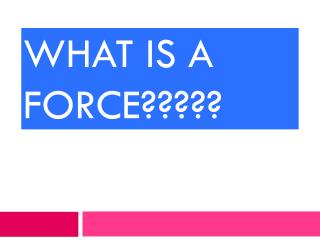# What is a force????? - PowerPoint PPT PresentationDownload PresentationWhat is a force?????

Presentation Description
Download Presentation## What is a force?????

- - - - - - - - - - - - - - - - - - - - - - - - - - - E N D - - - - - - - - - - - - - - - - - - - - - - - - - - -
##### Presentation Transcript

1. What is a force?????

2. PULL … or a pull

3. PUSH

4. PULL

5. FORCES NOT FORCES • Weight • Magnetism • Friction • Gravity • Thrust • Mass • Inertia • Speed • Acceleration • Velocity

6. GRAVITY MAGNETISM WEIGHT

7. FRICTION When an object moves in one direction, sliding past another object, friction moves it in the opposite direction!

8. THRUST Thrust is a strong push that quickly moves an object

9. Forces always occur in pairs, never alone

10. NET FORCE is the combining of ALL forces on an object • Each person is putting a force on the rope! • Combined, they have a net force!

11. Forces moving in opposite directions are subtracted 20N 10N NET FORCE ON THE CAR = 10 N

12. 30 N NET FORCE ON THE TOY = 15N 15 N

13. Forces moving in the same direction are added 12 N 12 N NET FORCE ON THE CAR = 24 N

14. Newton’s 1st Law of Motion Objects at rest remain at rest, objects in motion remain in motion, unless acted on by an unbalanced force.

15. What is a balanced force?

16. BALANCED FORCES • All forces acting on an object are equal and opposite in direction. • Net force equals zero • Causes no change in motion of the object

17. BALANCED FORCES 20 N 20 N NET FORCE = 0N NO MOVEMENT!!!!!

18. 20N 20N NET FORCE ON THE CAR= 0N

19. UNBALANCED FORCES • All forces acting on an object are NOT equal • The net force will NOT be 0 N • There WILL be a change in motion

20. The force of the boys pushing on the car, is greater than the force of friction. • The forces are unbalanced. 24 N FRICTION 4 N What is the NET FORCE? Which direction will the car move?

21. 10 N 50 N

22. Which way is this car going to move? 100 N 10 N

23. The car is in motion, until acted on by the wall. • The wall has more force than the car, so it will be stopped

24. This plane has a constant airspeed. • When a force (THRUST) is acted on it, the airspeed will increase, and the plane will accelerate. • If a different force (DRAG) is acted on it, the airspeed will decrease, and the plane will slow down.

25. The soccer ball remains at rest, until someone exerts thrust on it by kicking it. The pitcher throws a baseball in a certain pathway. The batter exerts a thrust on the ball with the bat, and the ball changes direction.

26. Newton’s 2nd Law of Motion Force (F) = mass (m) x acceleration (a)

27. Newton’s 3rd Law of Motion For every action, there is an equal and opposite reaction.